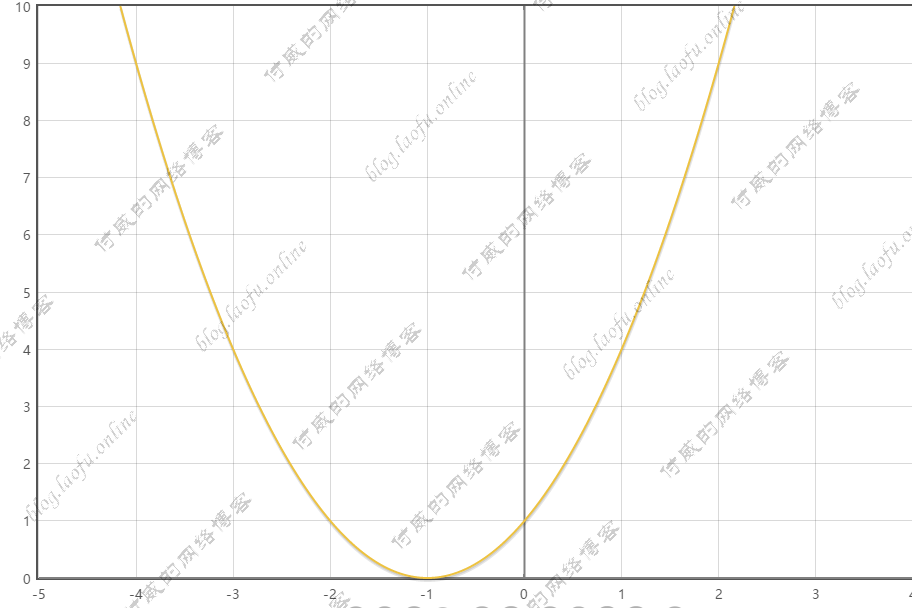# tensorflow的学习笔记--学习率

### 学习率

1次 参数w：5 5-0.2*(2*5+2)=2.6
2次 参数w：2.6 2.6-0.2*(2*2.6+2)=1.16
3次 参数w：1.16 1.16-0.2*(2*1.16+2)=0.296
4次 参数w：0.296
…..#coding:utf-8
# 设损失函数 loss=(w+1)^2 令 w初值是常数5，反向传播就是最优w,即求最小的loss对应的w值
import tensorflow as tf

#定义待优化参数w初值为5
w=tf.Variable(tf.constant(5,dtype=tf.float32))

# 定义损失函数loss
loss=tf.square(w+1)

with tf.Session() as sess:
init_op=tf.global_variables_initializer()
sess.run(init_op)

for i in range(40):
sess.run(train_step)
w_val=sess.run(w)
loss_val=sess.run(loss)
print("After %s steps: w is %f,loss is %f" %(i,w_val,loss_val))


  After 0 steps: w is 2.600000,loss is 12.959999
After 1 steps: w is 1.160000,loss is 4.665599
After 2 steps: w is 0.296000,loss is 1.679616
After 3 steps: w is -0.222400,loss is 0.604662
After 4 steps: w is -0.533440,loss is 0.217678
After 5 steps: w is -0.720064,loss is 0.078364
After 6 steps: w is -0.832038,loss is 0.028211
After 7 steps: w is -0.899223,loss is 0.010156
After 8 steps: w is -0.939534,loss is 0.003656
After 9 steps: w is -0.963720,loss is 0.001316
After 10 steps: w is -0.978232,loss is 0.000474
After 11 steps: w is -0.986939,loss is 0.000171
After 12 steps: w is -0.992164,loss is 0.000061
After 13 steps: w is -0.995298,loss is 0.000022
After 14 steps: w is -0.997179,loss is 0.000008
After 15 steps: w is -0.998307,loss is 0.000003
After 16 steps: w is -0.998984,loss is 0.000001
After 17 steps: w is -0.999391,loss is 0.000000
After 18 steps: w is -0.999634,loss is 0.000000
After 19 steps: w is -0.999781,loss is 0.000000
After 20 steps: w is -0.999868,loss is 0.000000
After 21 steps: w is -0.999921,loss is 0.000000
After 22 steps: w is -0.999953,loss is 0.000000
After 23 steps: w is -0.999972,loss is 0.000000
After 24 steps: w is -0.999983,loss is 0.000000
After 25 steps: w is -0.999990,loss is 0.000000
After 26 steps: w is -0.999994,loss is 0.000000
After 27 steps: w is -0.999996,loss is 0.000000
After 28 steps: w is -0.999998,loss is 0.000000
After 29 steps: w is -0.999999,loss is 0.000000
After 30 steps: w is -0.999999,loss is 0.000000
After 31 steps: w is -1.000000,loss is 0.000000
After 32 steps: w is -1.000000,loss is 0.000000
After 33 steps: w is -1.000000,loss is 0.000000
After 34 steps: w is -1.000000,loss is 0.000000
After 35 steps: w is -1.000000,loss is 0.000000
After 36 steps: w is -1.000000,loss is 0.000000
After 37 steps: w is -1.000000,loss is 0.000000
After 38 steps: w is -1.000000,loss is 0.000000
After 39 steps: w is -1.000000,loss is 0.000000


### 学习率的设置

After 1 steps: w is 5.000000,loss is 36.000000
After 2 steps: w is -7.000000,loss is 36.000000
....
After 37 steps: w is 5.000000,loss is 36.000000
After 38 steps: w is -7.000000,loss is 36.000000
After 39 steps: w is 5.000000,loss is 36.000000



global_step=tf.Variable(0,trainable=False)
learning_rate=tf.train.exponential_decay(LEARNINF_RATE_BASE,global_step,LEARNINF_RATE_STEP,LEARNINF_RATE_DECAY,staircase=True)


#coding:utf-8
# 设损失函数 loss=(w+1)^2 令 w初值是常数5，反向传播就是求最优w,即求最小的loss对应的w值
import tensorflow as tf

LEARNINF_RATE_BASE=0.1 # 初始学习率
LEARNINF_RATE_DECAY=0.99 # 学习率衰减率
LEARNINF_RATE_STEP=1 # 喂入多少轮BATCH_SIZE后，更新一次学习率，一般设为:总样本数/BATCH_SIZE

# 运行几轮BATCH_SIZE的计数器，初始值给0，设为不被训练
global_step=tf.Variable(0,trainable=False)

#定义指数下降学习率
learning_rate=tf.train.exponential_decay(LEARNINF_RATE_BASE,global_step,LEARNINF_RATE_STEP,LEARNINF_RATE_DECAY,staircase=True)

#定义待优化参数w初值为5
w=tf.Variable(tf.constant(5,dtype=tf.float32))

# 定义损失函数loss
loss=tf.square(w+1)

).minimize(loss,global_step=global_step)

with tf.Session() as sess:
init_op=tf.global_variables_initializer()
sess.run(init_op)

for i in range(40):
sess.run(train_step)
learning_rate_val=sess.run(learning_rate)
global_step_val=sess.run(global_step)
w_val=sess.run(w)
loss_val=sess.run(loss)
print("After %s ,global_step is %s,w is %f,learning_rate is %f,loss is %f" %(i,global_step_val,w_val,learning_rate_val,loss_val))



After 0 ,global_step is 1,w is 3.800000,learning_rate is 0.099000,loss is 23.040001
After 1 ,global_step is 2,w is 2.849600,learning_rate is 0.098010,loss is 14.819419
After 2 ,global_step is 3,w is 2.095001,learning_rate is 0.097030,loss is 9.579033
After 3 ,global_step is 4,w is 1.494386,learning_rate is 0.096060,loss is 6.221961
After 4 ,global_step is 5,w is 1.015167,learning_rate is 0.095099,loss is 4.060896
After 5 ,global_step is 6,w is 0.631886,learning_rate is 0.094148,loss is 2.663051
After 6 ,global_step is 7,w is 0.324608,learning_rate is 0.093207,loss is 1.754587
After 7 ,global_step is 8,w is 0.077684,learning_rate is 0.092274,loss is 1.161403
After 8 ,global_step is 9,w is -0.121202,learning_rate is 0.091352,loss is 0.772287
After 9 ,global_step is 10,w is -0.281761,learning_rate is 0.090438,loss is 0.515867
After 10 ,global_step is 11,w is -0.411674,learning_rate is 0.089534,loss is 0.346128
After 11 ,global_step is 12,w is -0.517024,learning_rate is 0.088638,loss is 0.233266
After 12 ,global_step is 13,w is -0.602644,learning_rate is 0.087752,loss is 0.157891
After 13 ,global_step is 14,w is -0.672382,learning_rate is 0.086875,loss is 0.107334
After 14 ,global_step is 15,w is -0.729305,learning_rate is 0.086006,loss is 0.073276
After 15 ,global_step is 16,w is -0.775868,learning_rate is 0.085146,loss is 0.050235
After 16 ,global_step is 17,w is -0.814036,learning_rate is 0.084294,loss is 0.034583
After 17 ,global_step is 18,w is -0.845387,learning_rate is 0.083451,loss is 0.023905
After 18 ,global_step is 19,w is -0.871193,learning_rate is 0.082617,loss is 0.016591
After 19 ,global_step is 20,w is -0.892476,learning_rate is 0.081791,loss is 0.011561
After 20 ,global_step is 21,w is -0.910065,learning_rate is 0.080973,loss is 0.008088
After 21 ,global_step is 22,w is -0.924629,learning_rate is 0.080163,loss is 0.005681
After 22 ,global_step is 23,w is -0.936713,learning_rate is 0.079361,loss is 0.004005
After 23 ,global_step is 24,w is -0.946758,learning_rate is 0.078568,loss is 0.002835
After 24 ,global_step is 25,w is -0.955125,learning_rate is 0.077782,loss is 0.002014
After 25 ,global_step is 26,w is -0.962106,learning_rate is 0.077004,loss is 0.001436
After 26 ,global_step is 27,w is -0.967942,learning_rate is 0.076234,loss is 0.001028
After 27 ,global_step is 28,w is -0.972830,learning_rate is 0.075472,loss is 0.000738
After 28 ,global_step is 29,w is -0.976931,learning_rate is 0.074717,loss is 0.000532
After 29 ,global_step is 30,w is -0.980378,learning_rate is 0.073970,loss is 0.000385
After 30 ,global_step is 31,w is -0.983281,learning_rate is 0.073230,loss is 0.000280
After 31 ,global_step is 32,w is -0.985730,learning_rate is 0.072498,loss is 0.000204
After 32 ,global_step is 33,w is -0.987799,learning_rate is 0.071773,loss is 0.000149
After 33 ,global_step is 34,w is -0.989550,learning_rate is 0.071055,loss is 0.000109
After 34 ,global_step is 35,w is -0.991035,learning_rate is 0.070345,loss is 0.000080
After 35 ,global_step is 36,w is -0.992297,learning_rate is 0.069641,loss is 0.000059
After 36 ,global_step is 37,w is -0.993369,learning_rate is 0.068945,loss is 0.000044
After 37 ,global_step is 38,w is -0.994284,learning_rate is 0.068255,loss is 0.000033
After 38 ,global_step is 39,w is -0.995064,learning_rate is 0.067573,loss is 0.000024
After 39 ,global_step is 40,w is -0.995731,learning_rate is 0.066897,loss is 0.000018



(本文完)